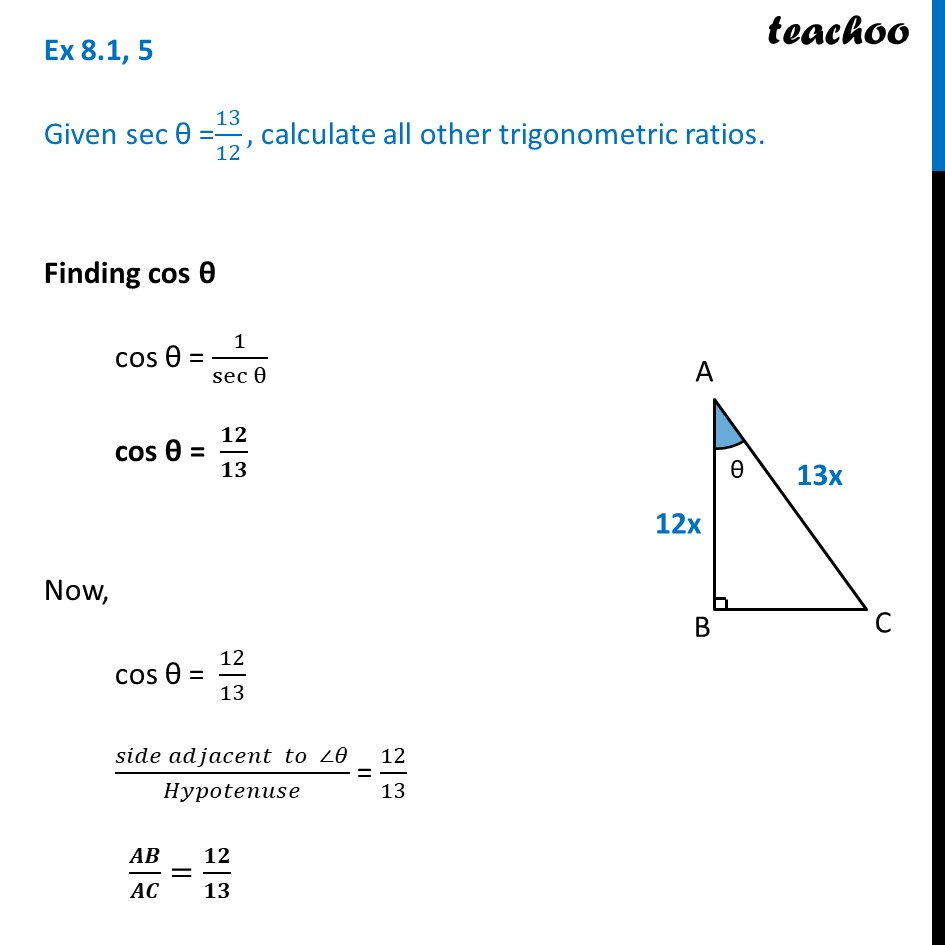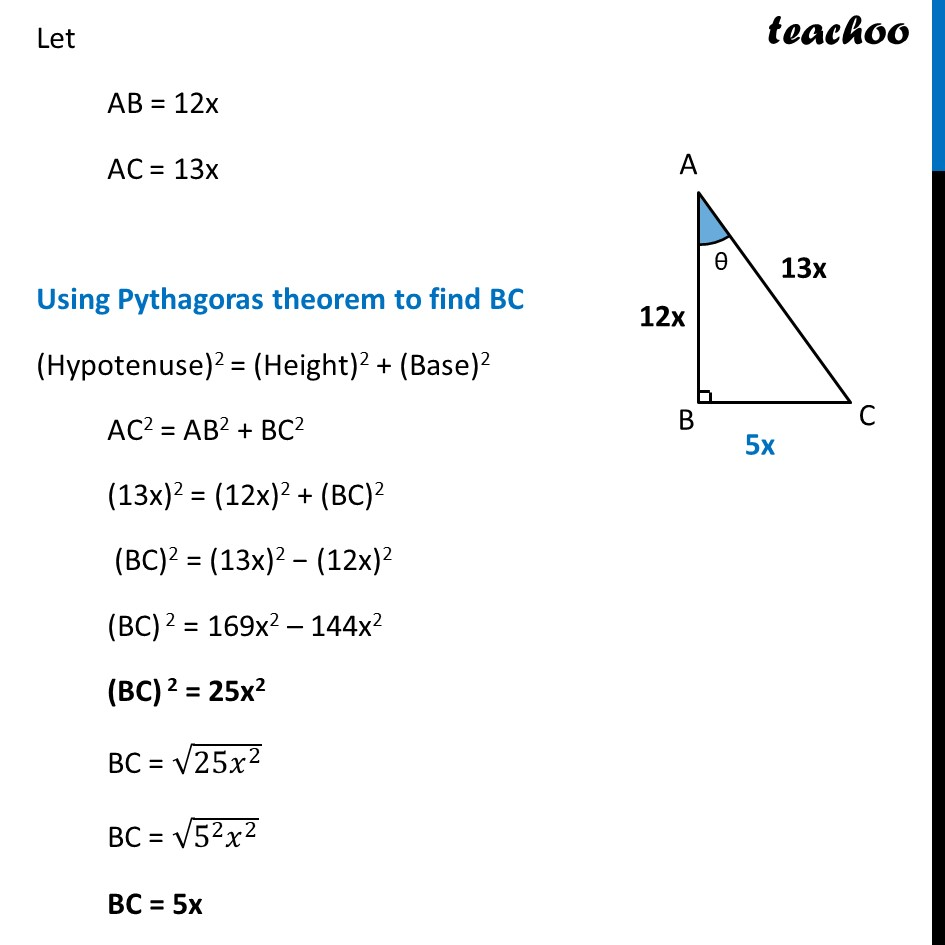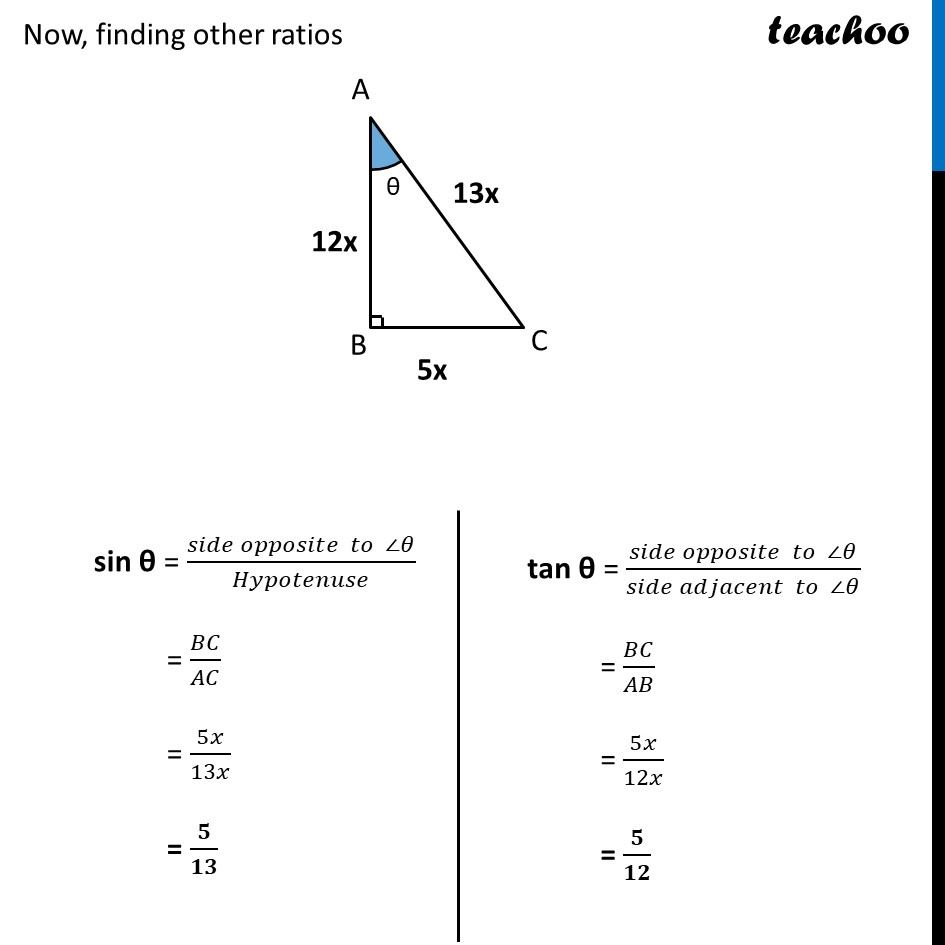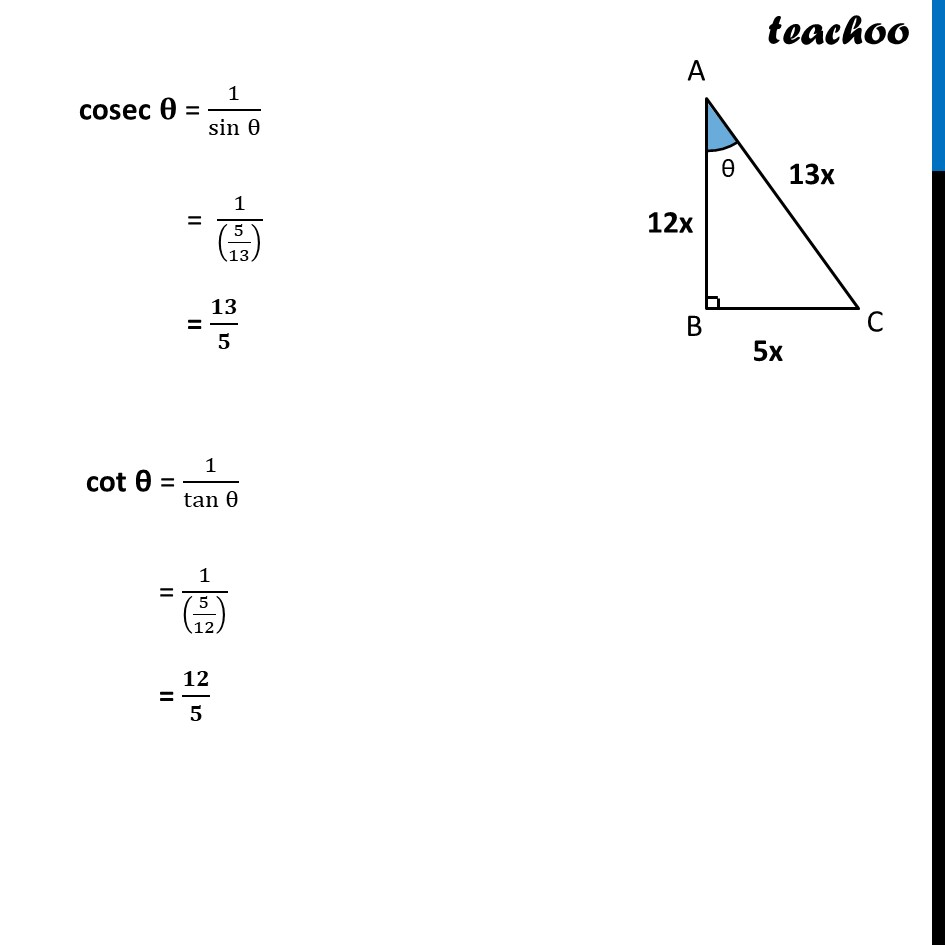Ex 8.1

Chapter 8 Class 10 Introduction to Trignometry
Serial order wiseLearn in your speed, with individual attention - Teachoo Maths 1-on-1 Class

### Transcript

Ex 8.1, 5 Given sec θ =13/12, calculate all other trigonometric ratios. Finding cos θ cos θ = 1/sec⁡θ cos θ = 𝟏𝟐/𝟏𝟑 Now, cos θ = 12/13 (𝑠𝑖𝑑𝑒 𝑎𝑑𝑗𝑎𝑐𝑒𝑛𝑡 𝑡𝑜 ∠𝜃)/𝐻𝑦𝑝𝑜𝑡𝑒𝑛𝑢𝑠𝑒 = 12/13 𝑨𝑩/𝑨𝑪=𝟏𝟐/𝟏𝟑 Let AB = 12x AC = 13x Using Pythagoras theorem to find BC (Hypotenuse)2 = (Height)2 + (Base)2 AC2 = AB2 + BC2 (13x)2 = (12x)2 + (BC)2 (BC)2 = (13x)2 − (12x)2 (BC) 2 = 169x2 – 144x2 (BC) 2 = 25x2 BC = √(25𝑥^2 ) BC = √(5^2 𝑥^2 ) BC = 5x Now, finding other ratios sin θ = (𝑠𝑖𝑑𝑒 𝑜𝑝𝑝𝑜𝑠𝑖𝑡𝑒 𝑡𝑜 ∠𝜃)/𝐻𝑦𝑝𝑜𝑡𝑒𝑛𝑢𝑠𝑒 = 𝐵𝐶/𝐴𝐶 = 5𝑥/13𝑥 = 𝟓/𝟏𝟑 tan θ = (𝑠𝑖𝑑𝑒 𝑜𝑝𝑝𝑜𝑠𝑖𝑡𝑒 𝑡𝑜 ∠𝜃)/(𝑠𝑖𝑑𝑒 𝑎𝑑𝑗𝑎𝑐𝑒𝑛𝑡 𝑡𝑜 ∠𝜃) = 𝐵𝐶/𝐴𝐵 = 5𝑥/12𝑥 = 𝟓/𝟏𝟐 cosec 𝛉 = 1/sin⁡θ = 1/((5/13) ) = 𝟏𝟑/𝟓 cot θ = 1/tan⁡θ = 1/((5/12) ) = 𝟏𝟐/𝟓